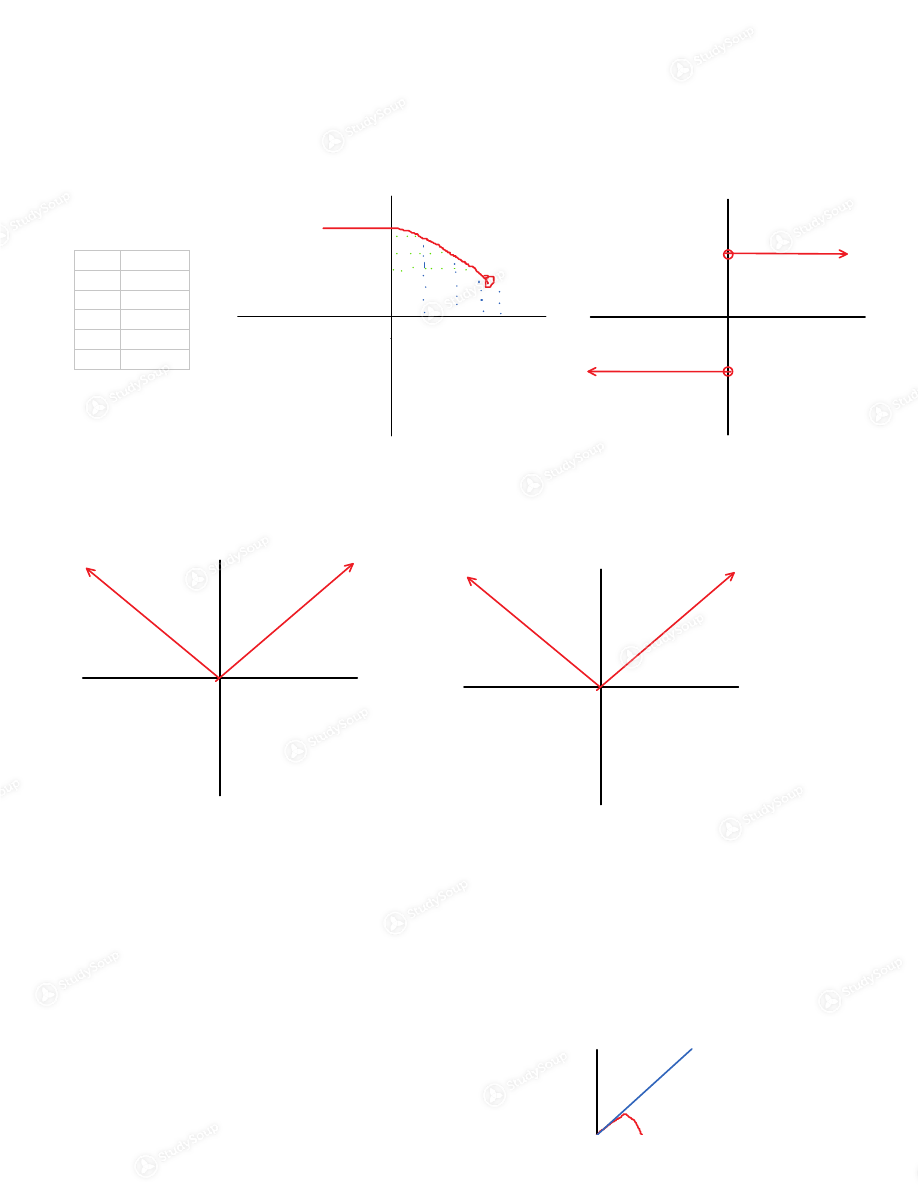Limited time offer 20% OFF StudySoup Subscription details

# UNLV - MATH 181 - Class Notes - Week 2

### Created by: Stephen Phillips Elite Notetaker

> > > > UNLV - MATH 181 - Class Notes - Week 2

UNLV - MATH 181 - Class Notes - Week 2

This preview shows page 1 of a 2 page document. to view the rest of the contentKey Idea: What distinguishes Calculus from other subjects (e.g. Algebra) is the concept of the  limit.
Limits are based on functions
Calculus therefore is defined by functions and limits. Definition: Intuitive - If the value of f(x) approaches the number L as x approaches a from the
left, we write:
lim 𝑓(𝑥) = 𝐿 𝒙 𝒇(𝒙) = 𝒚 𝑥 𝑓(𝑥 ) = 𝑦 𝑥 𝑓(𝑥 ) = 𝑦 𝑥 𝑓(𝑥 ) = 𝑦 --- --- a L 𝑓(𝑥 ) = 𝑦
𝑓(𝑥 ) = 𝑦
𝑓(𝑥 ) = 𝑦
𝑥        𝑥       𝑥 Example: Here we have one-sided limits, which is better than nothing,  but we still have a jump… lim 𝑓(𝑥) = 1 lim 𝑓(𝑥) = −1 1 Example: Better…the one-sided limits are the same, but no jumps lim 𝑓(𝑥) = 1 lim 𝑓(𝑥) = −1 …in which case, according to the definition of the limit lim 𝑓(𝑥) = 0 = lim 𝑓(𝑥) or lim 𝑓(𝑥) = − in which case lim 𝑓(𝑥) = 𝑓(0) Definition: A function f is continuous at x = a provided lim 𝑓(𝑥) = 𝑓(𝑎) F is defined at x = a: that is, 𝑎 ∈ D(f) (which means that f(a) is a number). 1. The limit exits 2. The limit equals the value of the function 3. Note: The definition is misleading. We must check 3 things: Polynomials at any point 1. Rational functions 2. Trigonometric functions 3. Exponential functions 4. Note: What types of functions are continuous? These: Example: 𝑎 ∈ 𝐷(𝑓). Let 𝑓(𝑥) = 2𝑥 − 3𝑥 + 4 lim 𝑓(𝑥) where a = 1 Evaluate limit using point continuity at 𝑎 = 1 ∈ 𝐷(𝑓) 1. lim 𝑓(𝑥) = 𝑓(1) = 3 Where
𝑓(1) = 2(1) − 3(1) + 4 = 3
𝑓 𝑓(𝑥) = 2𝑥 − 3𝑥 + 4 𝑋 = 𝐷(𝑓) = ℝ 𝑌 = ℝ Note: It is very misleading to write the equation as: lim 𝑥 − 1 𝑥 − 1 ⎯⎯⎯⎯⎯⎯= lim  𝑥 + 1 = (1) + 1 = 2 since these are two different functions! Example: Let 𝑓(𝑥) = 𝑥 + 1    𝑥 < 1 −2𝑥 + 4    𝑥 ≥ 1 Note: 𝑓 𝑥 = 𝑥 + 1    𝑥 < 1 −2𝑥 + 4    𝑥 ≥ 1 𝑋 = 𝐷 𝑓 = ℝ 1 f 𝒈 2. Limits and Continuity Monday, January 28, 2019 10:34 AM     Chapter 1 - Functions and Limits Page 1

This is the end of the preview. Please to view the rest of the contentJoin more than 18,000+ college students at University of Nevada - Las Vegas who use StudySoup to get ahead
##### Description: These cover the second section of Chapter 1.
2 Pages 54 Views 43 Unlocks
• Better Grades Guarantee
• 24/7 Homework help
• Notes, Study Guides, Flashcards + More!
Get Full Access to UNLV - Class Notes - Week 2
×
Get Full Access to UNLV - Class Notes - Week 2

I don't want to reset my password

Need help? Contact support

Need an Account? Is not associated with an account
We're here to help

Having trouble accessing your account? Let us help you, contact support at +1(510) 944-1054 or support@studysoup.com

Password Reset Request Sent An email has been sent to the email address associated to your account. Follow the link in the email to reset your password. If you're having trouble finding our email please check your spam folder
Already have an Account? Is already in use